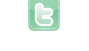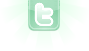how to make actor bounce around world like a ball

what I need is code to make my actor randomly move in a direction once run is clicked and for that actor to hit the walls of my world and bounce off of them like a ball. world size is 850,480 and the actor is also an enemy that eats the player if that helps in anyway
To make it use a random direction at the beginning, set the rotation randomly in the constructor of the class. Then in its act method, just move straight, check if it is touching a wall and if so, change the rotation. This is usually done by splitting the X and Y movement, so you can decide where to turn after the intersection with a wall.
i have no idea how to code the part where the actor touches the walls and bounces off realistically because i am very new to greenfoot, is there any way i can get help with this?
(for example)
```public class Enemy extends Actor {
public Enemy(){
setRotation(Greenfoot.getRandomNumber(360));
}
int dx = 2;
int dy = 2;

public void act(){
if (getX() < 0){
dx = 2;
}
if (getX() + getImage().getWidth() > getWorld().getWidth()){
dx = -2;
}
if (getY() < 0){
dy = 2;
}
if (getY() + getImage().getHeight() > getWorld().getHeight()){
dy = -2;
}
setLocation(getX() + dx, getY() + dy);
}
}```
such an amazing example it helped a lot and thank you, but two things, all the enemies go the same way from the start (to the bottom right)and they have seem to stop eating the player, am i missing something?
```import greenfoot.*;
public class Jelly extends Actor
{
private int count = 2;
public  void enemy()
{
setRotation(Greenfoot.getRandomNumber(360));
}
int dx = 2;
int dy = 2;

public void act()
{
if (getX() <= 5 || getX() >= getWorld().getWidth() - 5)
{
dx = 2;
}
if (getX() + getImage().getWidth() > getWorld().getWidth())
{
dx = -2;
}
if (getY() <= 5 || getY() >= getWorld().getHeight() - 5)
{
dy = 2;
}
if (getY() + getImage().getHeight() > getWorld().getHeight())
{
dy = -2;
}
setLocation(getX() + dx, getY() + dy);
}
public void eat()
{
Actor jelly;
jelly = getOneObjectAtOffset(0, 0, Shark.class);
if (jelly != null)
{
World world;
world = getWorld();
world.removeObject(jelly);
```public Jelly()